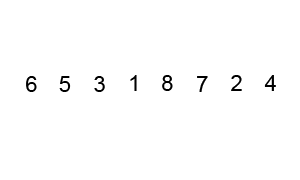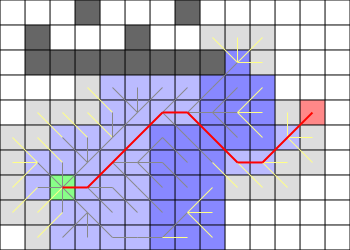# Algorithmic cheatsheet

2014-10-02

This page sums up some important results from computer science. They are extracted from the Introduction to Algorithms (Third Edition), by Thomas H. Cormen, Charles E. Leiserson, Ronald L. Rivest and Clifford Stein. We highly recommend it.

The following information is organized in several sections grouping definitions. Each definition points to the Introduction to Algorithms for further information using the abbreviation CLRS (from the authors’ names).

The formulæ are written using $$\LaTeX$$ and the final render of the page is done by the Javascript library MathJax (currently the simplest way to use $$\LaTeX$$ in HTML).

The current figures use images from external websites. Clicking on it will redirect you to their original webpages.

# General results

## Master Theorem

CLRS: p93

Recurrence of the form: $$T(n) = a T(n/b) + O(f(n))$$

Case $$f(n) = O(\dots)$$ $$T(n) = O(\dots)$$
1 $$n^{\log_b a - \varepsilon}$$ $$n^{\log_b a}$$
2 $$n^{\log_b a}$$ $$\log n \times n^{\log_b a}$$
3 $$n^{\log_b a + \varepsilon}$$ and $$\exists c, a f(n/b) \leq c f(n)$$ $$f(n)$$

call tree; $$b$$ defines the maximal depth of the recursive calls, $$a$$ defines the maximal number of calls to $$f$$ at each level, summing the complexity of each of the $$O(n^{\log_b a})$$ calls to $$f$$, which depends on the depth, explains the cases of the master theorem (the illustraction come from the CLRS Second Edition)

## Matroid

CLRS: p437

Pair $$(S, \mathcal I)$$ with:

• $$S$$ is a finite set
• $$\emptyset \neq \mathcal I \subseteq \mathcal P(S)$$ (independent subsets)
• $$\forall A \subseteq B \in \mathcal I, A \in \mathcal I$$ (heredity)
• $$\forall A,B \in \mathcal I, |A| \lt |B| \Rightarrow \exists x \in B \backslash A, A \cup \{x\} \in \mathcal I$$(exchange)

## Greedy algorithms from matroids

CLRS: p439

$$(S, \mathcal I)$$ is a matroid. $$w$$ is a weight function over $$S$$. Greedy algorithm → choosing iteratively an element maximizing $$w$$:

• $$\{x\} \in \mathcal I$$ with maximum weight
• $$x$$ is in any independent subset with maximum weight
• $$\mathcal I' = \{B \subseteq S \backslash \{x\} : B \cup \{x\} \in \mathcal I\}$$ (subsets containing $$x$$, ignoring $$x$$)
• $$S' = \{y \in S, \{x,y\} \in \mathcal I)$$ (restricting elements)
• iterate on $$(S', \mathcal I')$$ (also a matroid)

# Data structures

## Heap

CLRS: p151

Sorted data structure (priority queue) Min-heap: parent is bigger than its children Max-heap: parent is bigger than its children

Operation Complexity
Memory use $$O(n)$$
Insert $$O(\log n)$$
Maximum $$O(1)$$
Extract-Max $$O(\log n)$$
Increase-Key $$O(\log n)$$

## Hash Table

CLRS: p256

Arbitrarily indexed table $$h$$ is a hash function to $$[1..m]$$

• Space: $$O(m)$$
• Locate: $$O(1 + n/m)$$

Note: Locate finds a location in the array where the user can read or write as usual.

## Binary Search Tree (BST)

CLRS: p286

Sorted data structure $$h$$ is the height of the tree

• Space: $$O(n)$$
• Search: $$O(h)$$
• Insert: $$O(h)$$
• Delete: $$O(h)$$

## Red-Black Tree

CLRS: p308

BST maintaining $$h = \log n$$ with:

• every node is either black (“normal”) or red (to be updated);
• the root is black;
• leaves are black;
• red nodes cannot have a red parent → there are no more red nodes than black ones;
• all simple paths from the root to a leaf have the same number of black nodes, limiting $$h$$.any simple path from the root to a leaf has 3 black nodes, there are no more red nodes than black ones. (source)

## B-Tree

CLRS: p484

Variant of the BST using a large fan-out $$t$$ to reduce disk-accesses Search, insert and delete take $$O(\log_t n)$$ disk accesses and $$O(t \log_t n)$$ CPU time.the fan-out is usually around 1000, making two levels enough most of the time (source)

## Union-find

CLRS: p568

Data structure for sets, supporting union of sets and finding the representative $$m$$ is the number of previously run Make-Set operations. $$\alpha$$ is the Ackerman function ($$\alpha(m) \leq 4$$ in practise).

• Make-Set: $$O(1)$$
• Union: $$O(1)$$
• Find-Set: $$O(\alpha(m))$$

http://scienceblogs.com/goodmath/2007/09/06/graph-searches-and-disjoint-se/

# Sorting

## Bubblesort

CLRS: p40 (problem 2-2) Complexity: $$O(n^2)$$

Fixing inverted adjacent values $$n$$ timesthe red squares highlight the currently checked pairs, all pairs have to be checked $$n$$ times (source)

## Insertion sort

CLRS: p16 (correctness), p24 (complexity) Complexity: $$O(n^2)$$

Inserting each element in a new array at the right location

## Merge sort

CLRS: p29 Complexity: $$O(n \log n)$$

Divide-and-conquer (sort each half, then merge)

## Heapsort

CLRS: p159 Complexity: $$O(n \log n)$$

Building a heap on the $$n$$ elements and extracting them all

## Quicksort

CLRS: p170 Complexity: $$O(n \log n)$$

Divide-and-conquer (choose a pivot, then filter lower and greater values)the elements are split in two sub-arrays using pivot as the limit value and each part is sorted recursively (source)

# Graph algorithms

## Kruskal

CLRS: p631 Complexity: $$O(E \log V)$$

Finding a minimum spanning tree Consider every edge in nondecreasing order, add to current forest if links two components. The components are handled using union-find.

## Prim

CLRS: p634 Complexity: $$O(E \log V)$$ (binary heap) Complexity: $$O(E + V \log V)$$ (Fibonacci heap)

Finding a minimum spanning tree Iteratively extract closest vertex $$u$$ and relax all edges $$(u, v)$$. The set of vertices is handled using a priority queue.

## Bellman-Ford

CLRS: p651 Complexity: $$O(E V)$$

Finding all shortest paths from a single source Just relax every edge of the graph |V| times Detects negative weight cycles

## Shortest paths from DAG

CLRS: p655 Complexity: $$O(V+E)$$ (including sorting)

Finding all shortest paths from a single source in Directed Acyclic Graphs Consider every vertex $$u$$ in topological order and relax all edges $$(u, v)$$

## Dijkstra

CLRS: p658 Complexity: $$O(V^2 + E)$$ (array) Complexity: $$O((E+V) \log V)$$ (binary heap) Complexity: $$O(V \log V + E)$$ (Fibonacci heap)

Finding all shortest paths from a single source with positive weights Iteratively extract closest vertex $$u$$ and relax all edges $$(u, v)$$. The set of vertices is handled using a priority queue.

## Floyd-Warshall

CLRS: p693 Complexity: $$O(V^3)$$

Finding the shortest path from all vertex pairs Relaxing pair distance $$|V|$$ times

## The Path-Finding Problemwe want to find a shortest or near-shortest path; Breadth First Search works well on unweighted graph (no water); Dijkstra generalize it to weighted graph; A* is a common path finding used in games which generalizes Dijkstra and Best First Search